John Szynal

•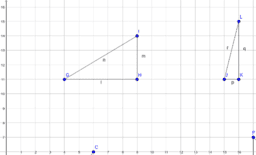Pythagorean Theorem Investigation

Activity

John Szynal

•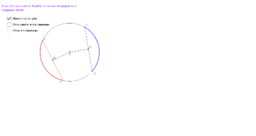Congruent Chords

Activity

John Szynal

•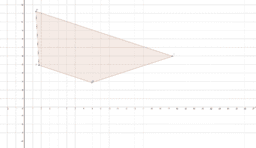Trapezoid

Activity

John Szynal

•Slope Field Logistical Growth

Activity

John Szynal

•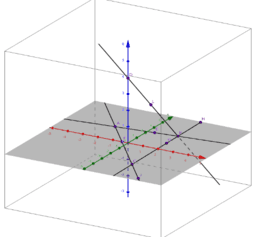Points, Lines, and Planes

Activity

John Szynal

•Where might systems come from?

Activity

John Szynal

•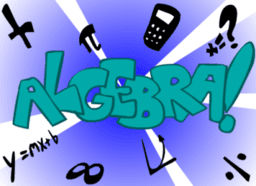Algebra I Activities

Book

John Szynal

•Lamp Post Problem

Activity

John Szynal

•Logistic Growth

Activity

John Szynal

•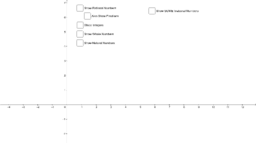Classifying Numbers with Sliders

Activity

John Szynal

•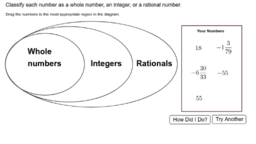Classifying Numbers Activity

Activity

John Szynal

•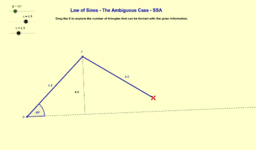Copy of Ambiguous Case SSA

Activity

John Szynal

•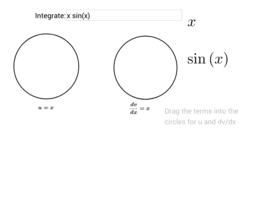Integration by Parts

Activity

John Szynal

•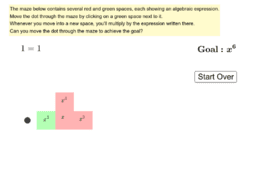Exponents Game

Book

John Szynal

•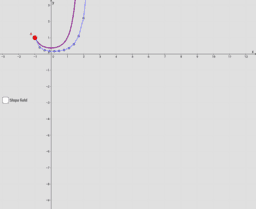Euler's Method Demonstration

Activity

John Szynal

•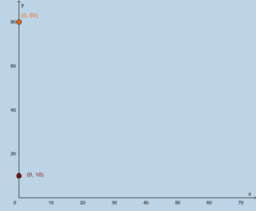System of Equation Story Problem Template

Activity

John Szynal

•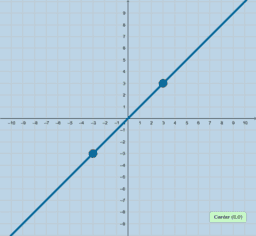Graphing Linear Inequalities (Slope Intercept)

Activity

John Szynal

•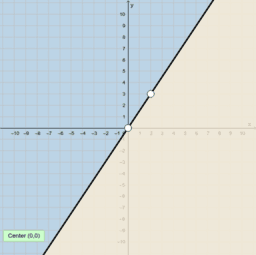Graphing Linear Inequalities (Standard Form)

Activity

John Szynal

•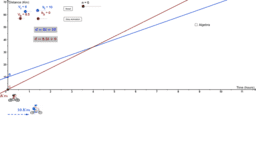Bicycle Race Problem

Activity

John Szynal

•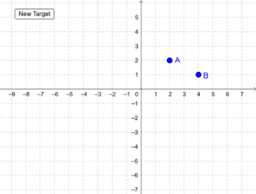Writing Equations of Lines

Activity

John Szynal

•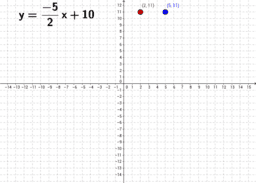Slope Intercept and Standard Form

Activity

John Szynal

•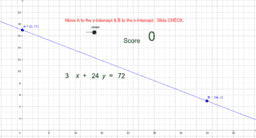Standard Form Practice

Activity

John Szynal

•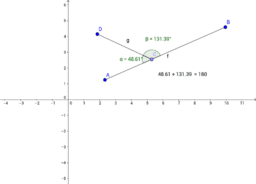Szynal Unit 2 Project

Book

John Szynal

•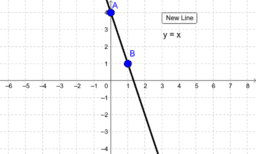Activity

John Szynal